# RS Aggarwal Solutions for Class 9 Maths Chapter 16: Presentation of Data in Tabular Form

The 16th chapter of RS Aggarwal Solutions for Class 9 explains the presentation of data and frequency distribution in tabular form. The solutions are prepared by highly experienced faculties having vast subject knowledge. The subject experts at BYJU’S design solutions in such a way it matches the understanding level of the students with 100% accuracy. The students can download the PDF and use it as reference material while solving the problems.

All the solutions of Chapter 16 are based on the CBSE syllabus and guidelines to help students in solving the problems easily. The students can also obtain chapter-wise solutions for the RS Aggarwal textbook to boost their exam preparation. RS Aggarwal Solutions for Class 9 Chapter 16 Presentation of Data in Tabular Form are provided here.

## RS Aggarwal Solutions for Class 9 Maths Chapter 16: Presentation of Data in Tabular Form Download PDF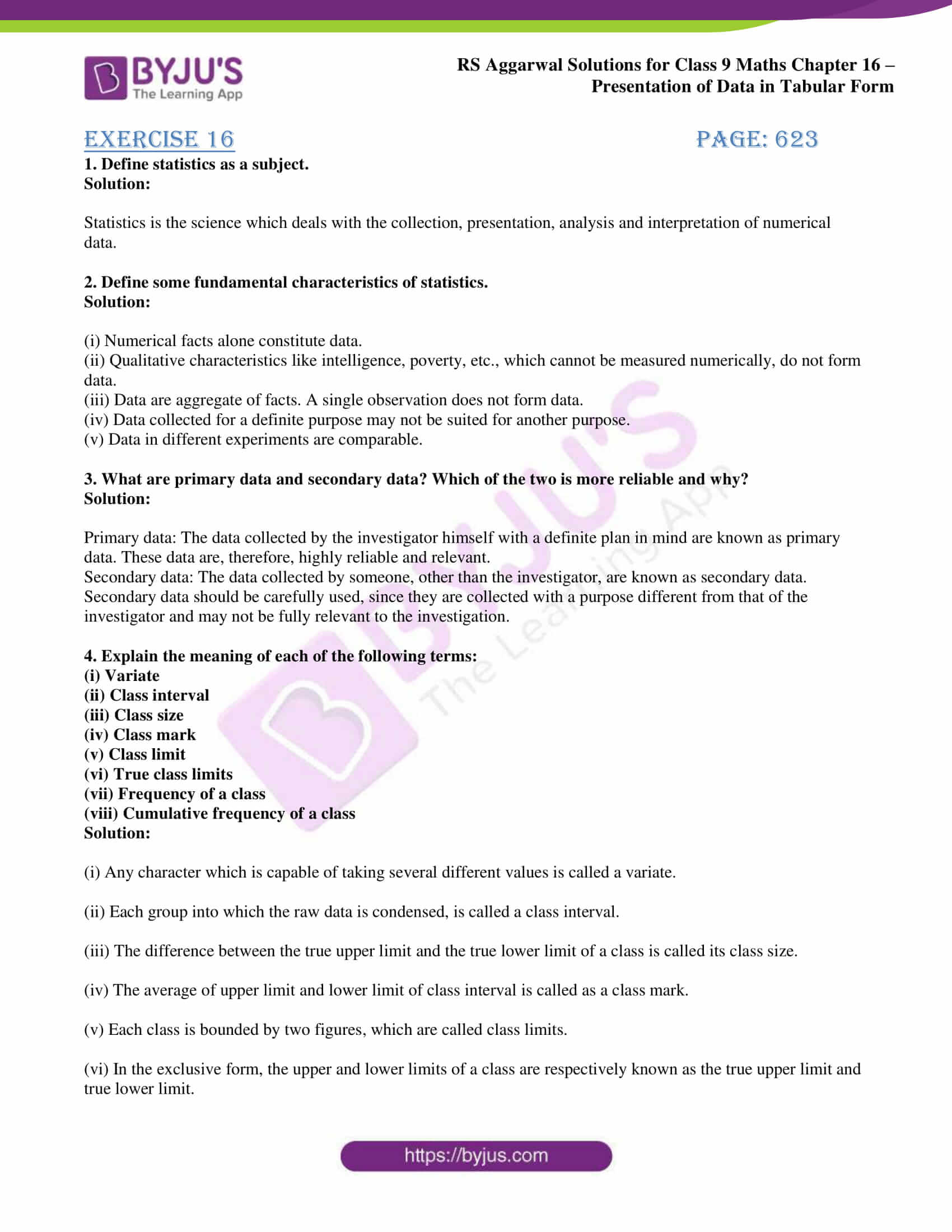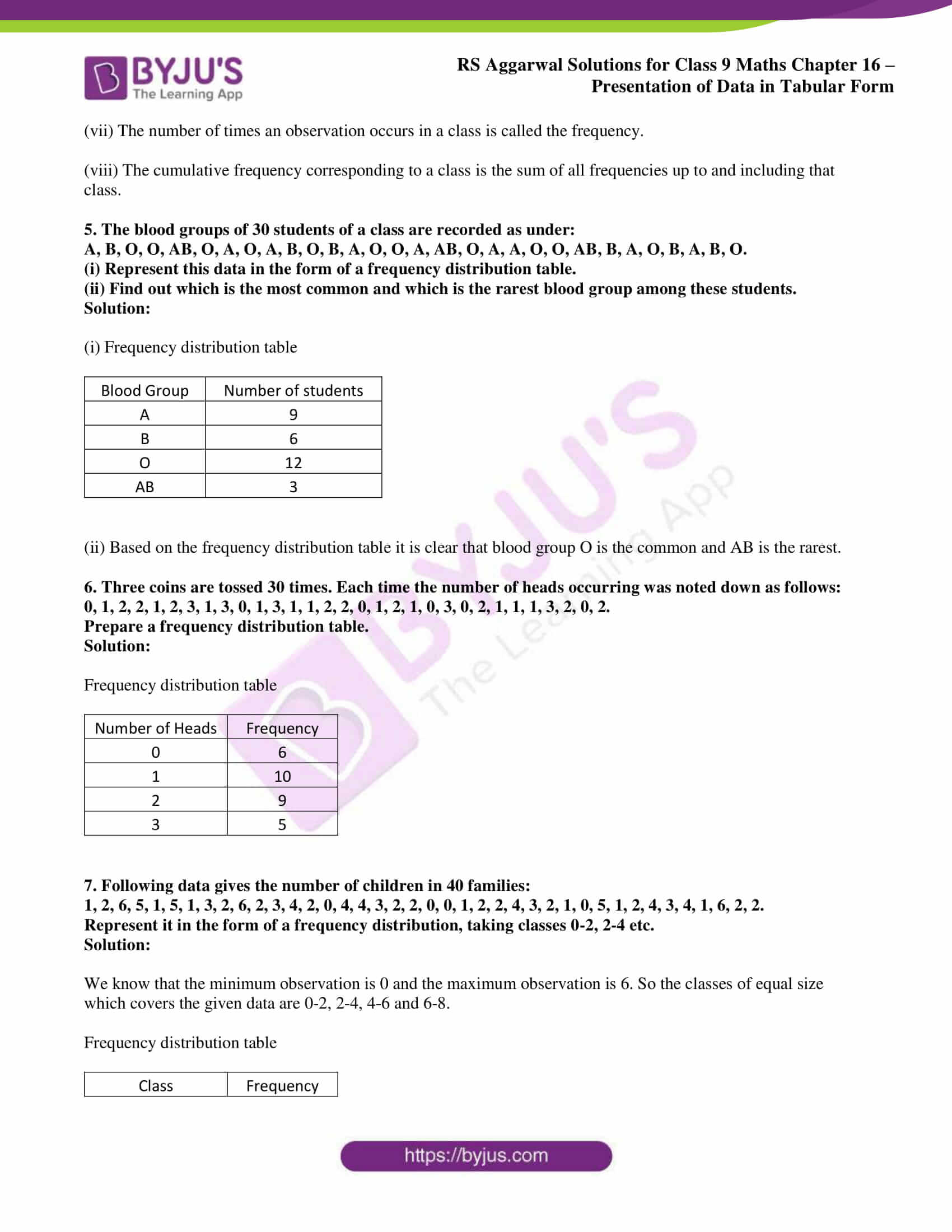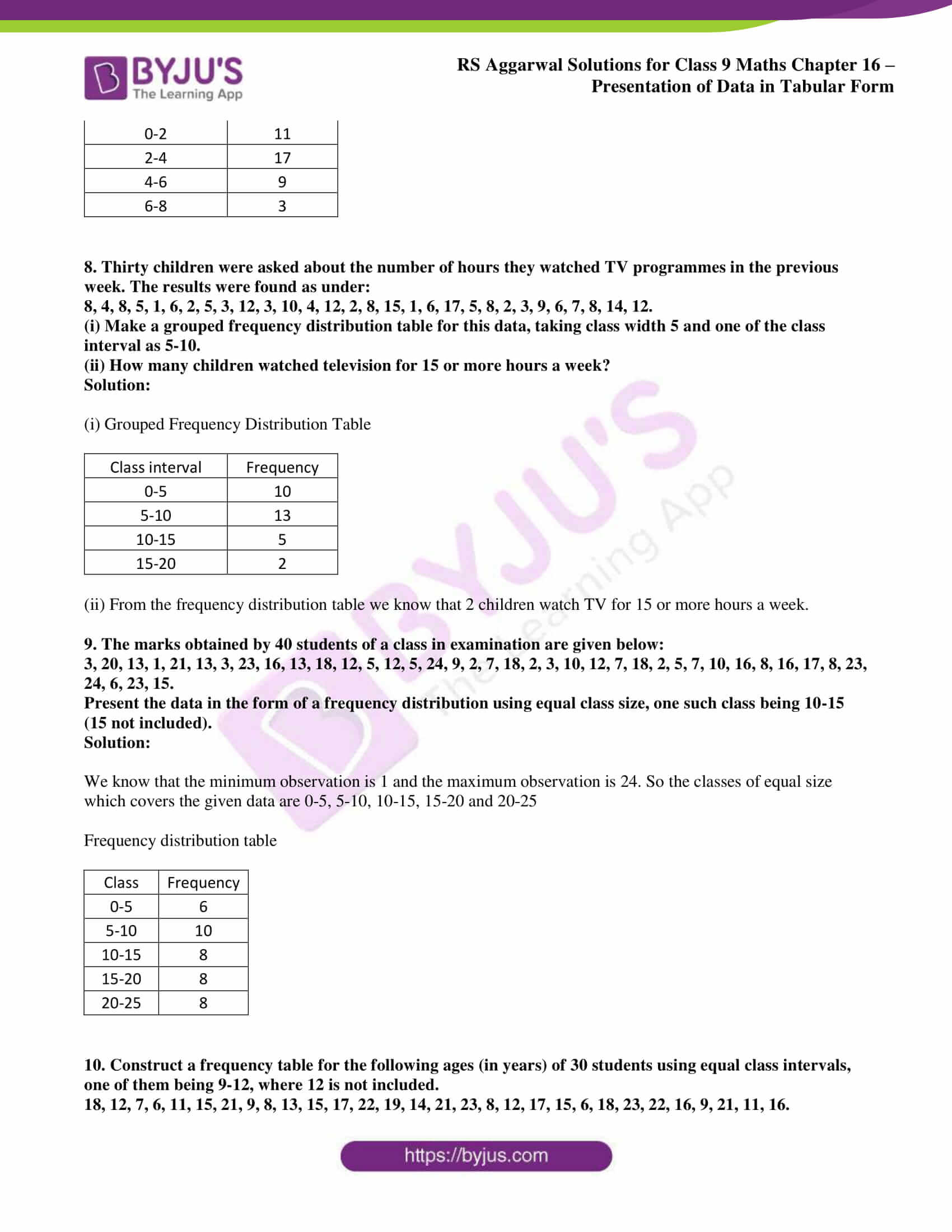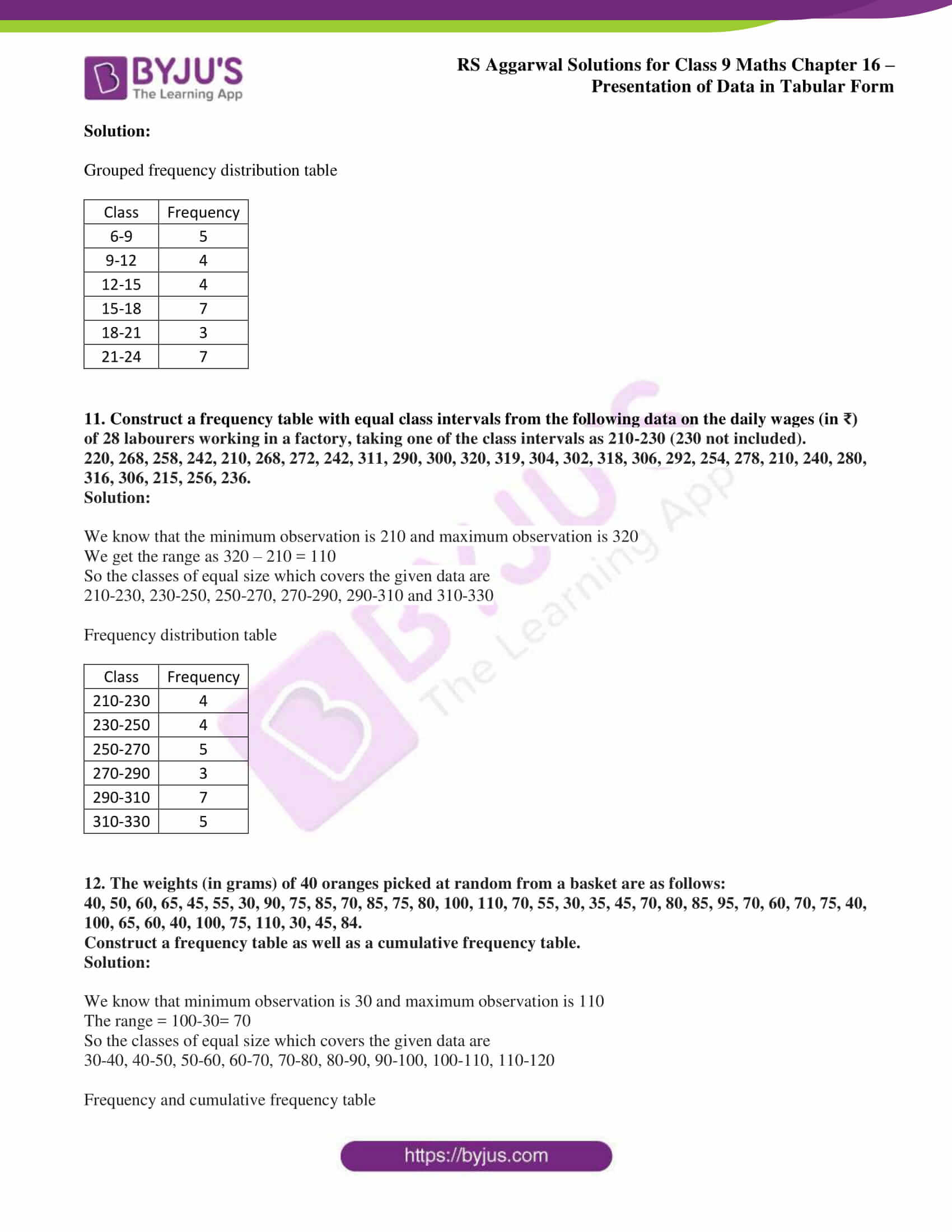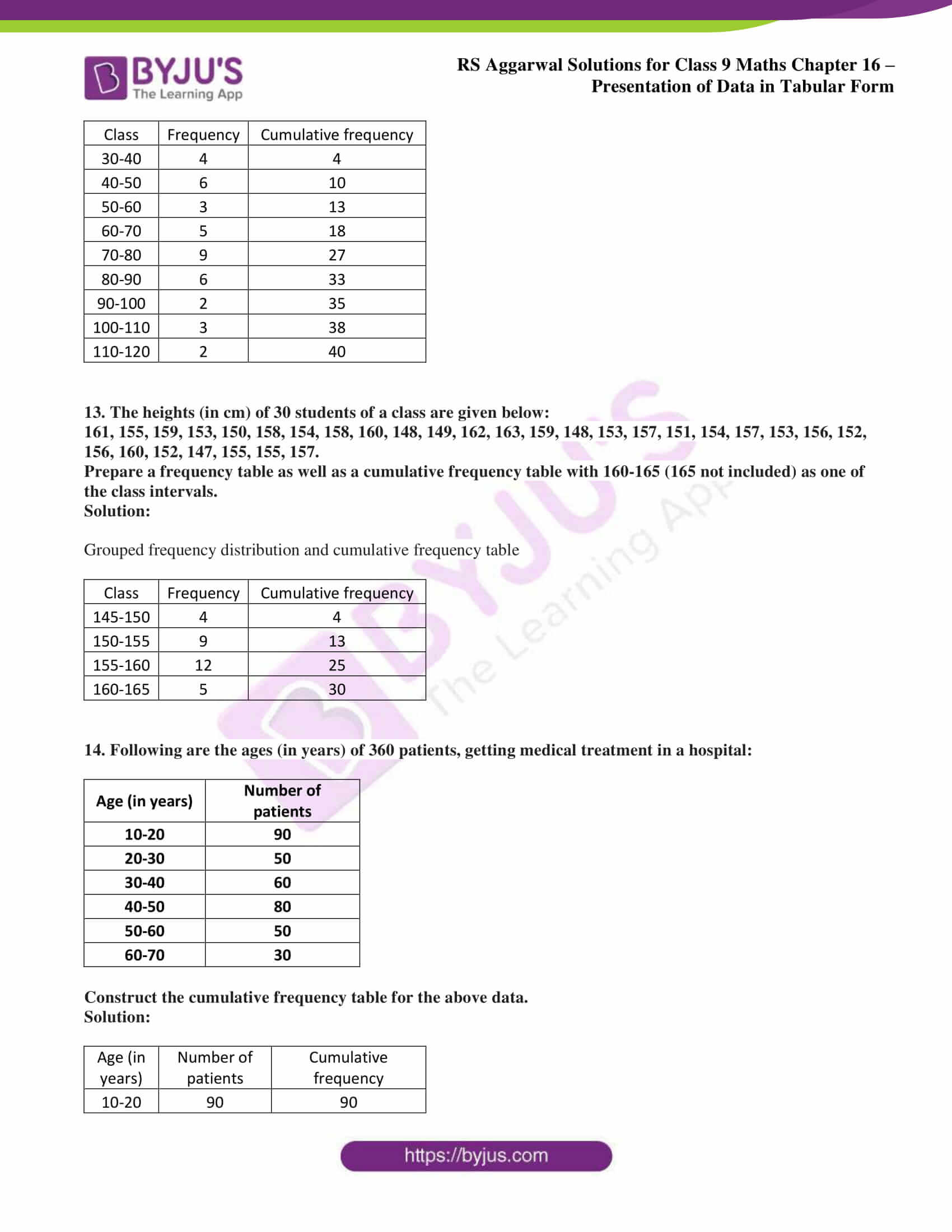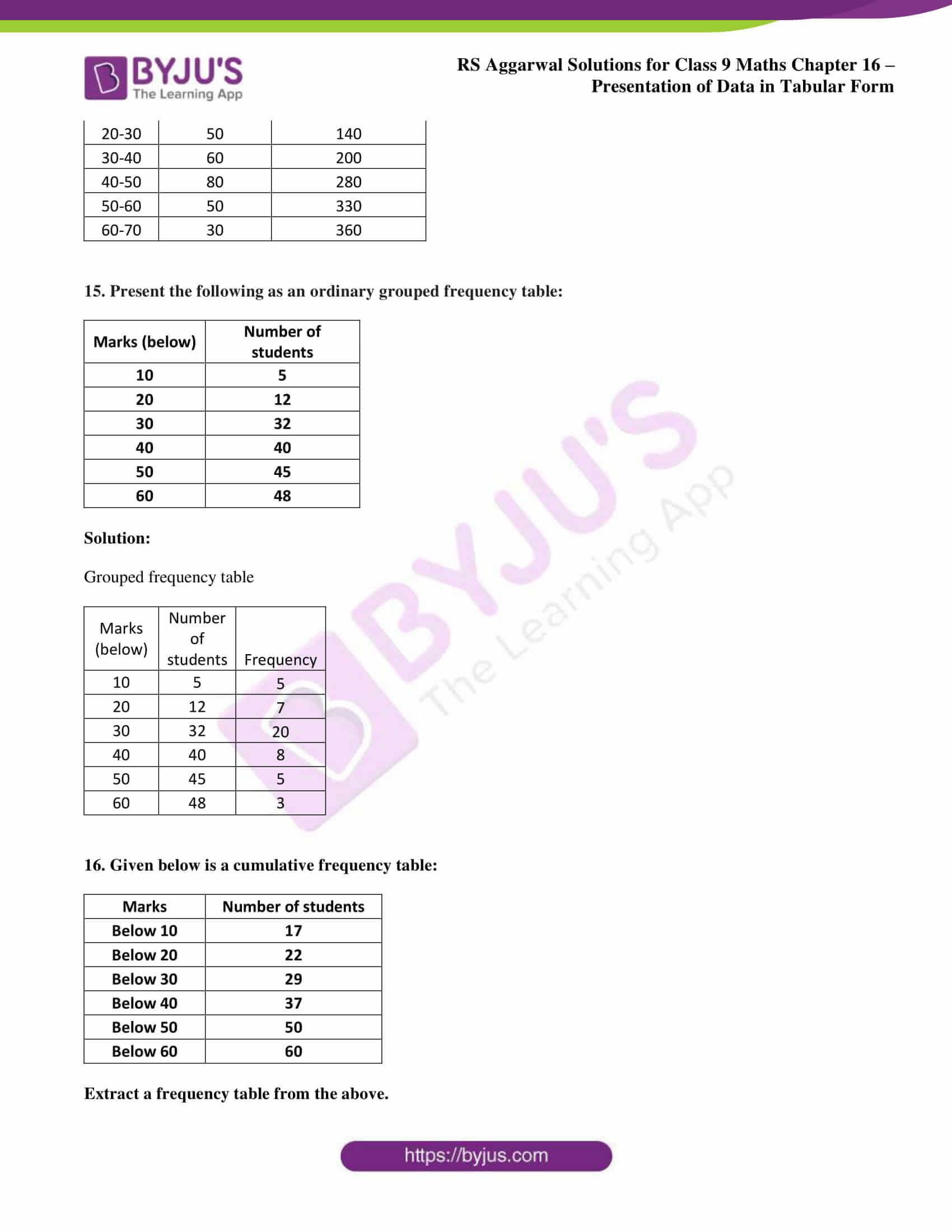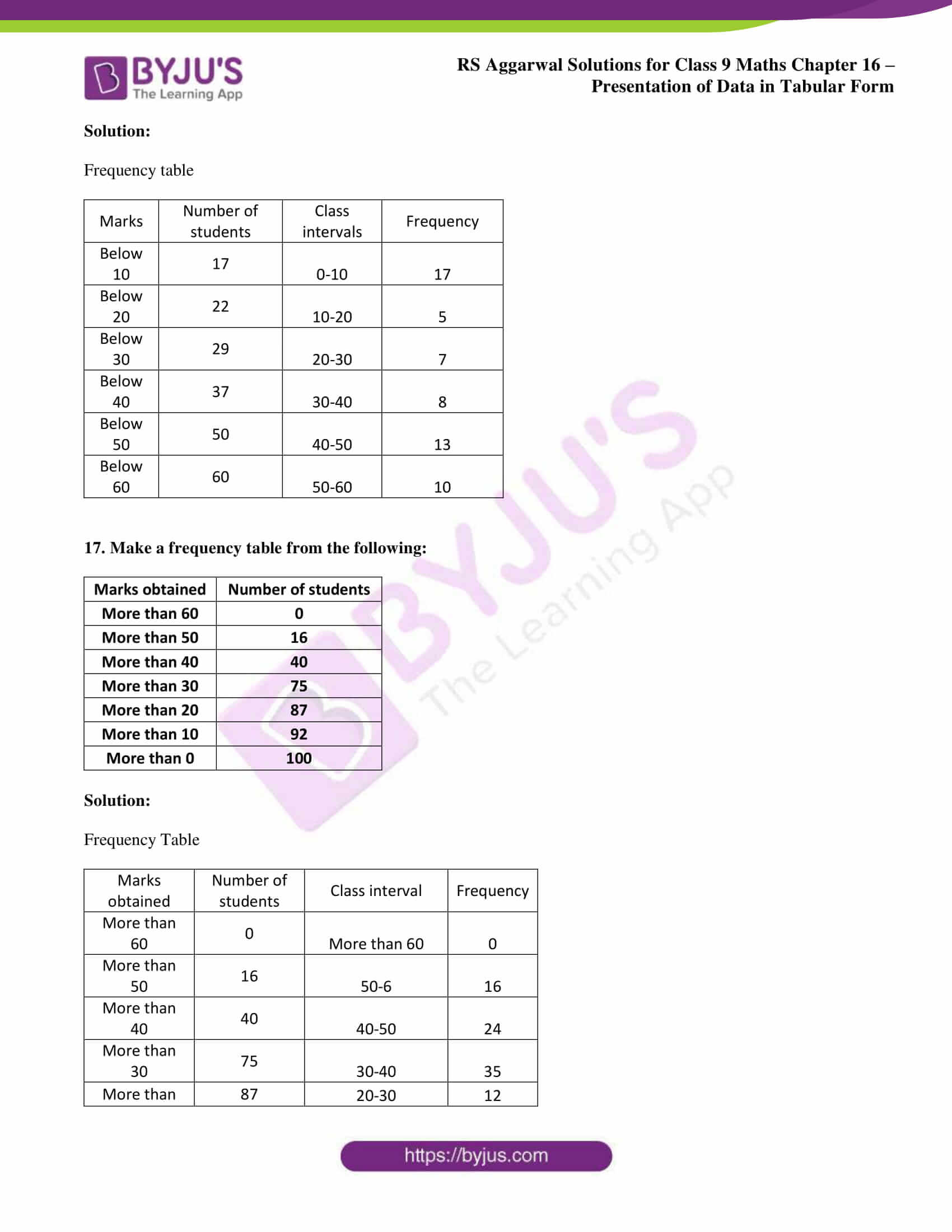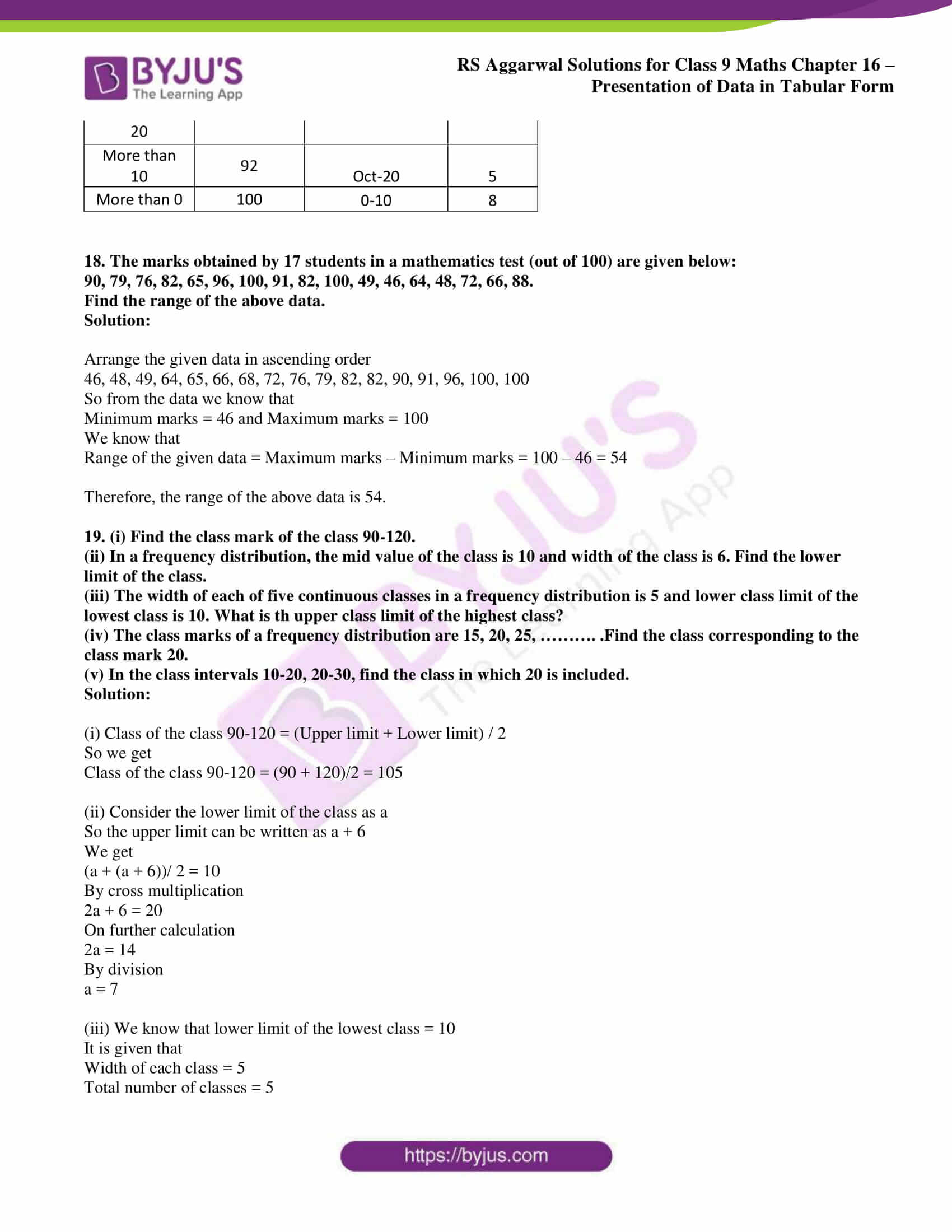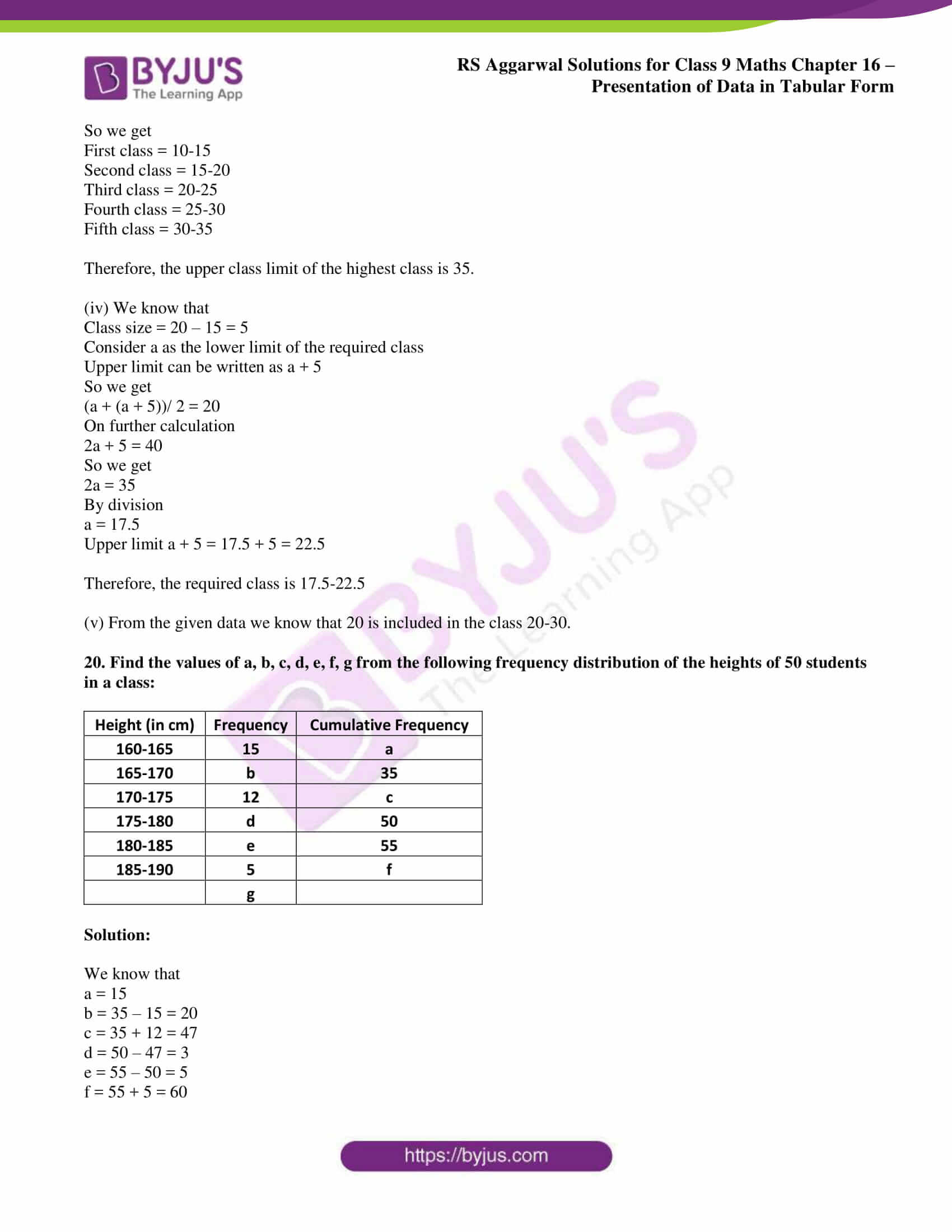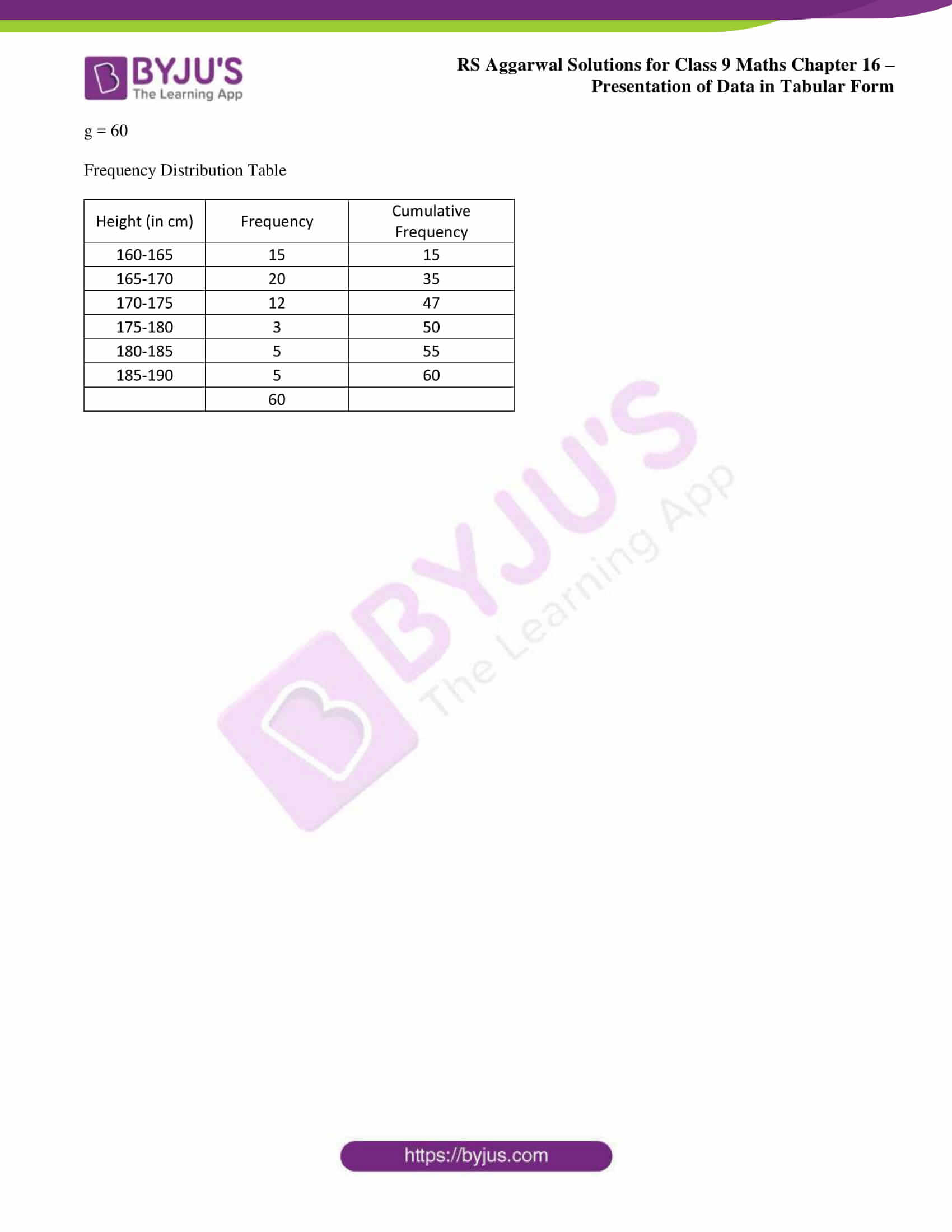## Access RS Aggarwal Solutions for Class 9 Maths Chapter 16: Presentation of Data in Tabular Form

Exercise 16 page: 623

1. Define statistics as a subject.

Solution:

Statistics is the science which deals with the collection, presentation, analysis and interpretation of numerical data.

2. Define some fundamental characteristics of statistics.

Solution:

(i) Numerical facts alone constitute data.

(ii) Qualitative characteristics like intelligence, poverty, etc., which cannot be measured numerically, do not form data.

(iii) Data are aggregate of facts. A single observation does not form data.

(iv) Data collected for a definite purpose may not be suited for another purpose.

(v) Data in different experiments are comparable.

3. What are primary data and secondary data? Which of the two is more reliable and why?

Solution:

Primary data: The data collected by the investigator himself with a definite plan in mind are known as primary data. These data are, therefore, highly reliable and relevant.

Secondary data: The data collected by someone, other than the investigator, are known as secondary data. Secondary data should be carefully used, since they are collected with a purpose different from that of the investigator and may not be fully relevant to the investigation.

4. Explain the meaning of each of the following terms:

(i) Variate

(ii) Class interval

(iii) Class size

(iv) Class mark

(v) Class limit

(vi) True class limits

(vii) Frequency of a class

(viii) Cumulative frequency of a class

Solution:

(i) Any character which is capable of taking several different values is called a variate.

(ii) Each group into which the raw data is condensed, is called a class interval.

(iii) The difference between the true upper limit and the true lower limit of a class is called its class size.

(iv) The average of upper limit and lower limit of class interval is called as a class mark.

(v) Each class is bounded by two figures, which are called class limits.

(vi) In the exclusive form, the upper and lower limits of a class are respectively known as the true upper limit and true lower limit.

(vii) The number of times an observation occurs in a class is called the frequency.

(viii) The cumulative frequency corresponding to a class is the sum of all frequencies up to and including that class.

5. The blood groups of 30 students of a class are recorded as under:

A, B, O, O, AB, O, A, O, A, B, O, B, A, O, O, A, AB, O, A, A, O, O, AB, B, A, O, B, A, B, O.

(i) Represent this data in the form of a frequency distribution table.

(ii) Find out which is the most common and which is the rarest blood group among these students.

Solution:

(i) Frequency distribution table

 Blood Group Number of students A 9 B 6 O 12 AB 3

(ii) Based on the frequency distribution table it is clear that blood group O is the common and AB is the rarest.

6. Three coins are tossed 30 times. Each time the number of heads occurring was noted down as follows:

0, 1, 2, 2, 1, 2, 3, 1, 3, 0, 1, 3, 1, 1, 2, 2, 0, 1, 2, 1, 0, 3, 0, 2, 1, 1, 1, 3, 2, 0, 2.

Prepare a frequency distribution table.

Solution:

Frequency distribution table

 Number of Heads Frequency 0 6 1 10 2 9 3 5

7. Following data gives the number of children in 40 families:

1, 2, 6, 5, 1, 5, 1, 3, 2, 6, 2, 3, 4, 2, 0, 4, 4, 3, 2, 2, 0, 0, 1, 2, 2, 4, 3, 2, 1, 0, 5, 1, 2, 4, 3, 4, 1, 6, 2, 2.

Represent it in the form of a frequency distribution, taking classes 0-2, 2-4 etc.

Solution:

We know that the minimum observation is 0 and the maximum observation is 6. So the classes of equal size which covers the given data are 0-2, 2-4, 4-6 and 6-8.

Frequency distribution table

 Class Frequency 0-2 11 2-4 17 4-6 9 6-8 3

8. Thirty children were asked about the number of hours they watched TV programmes in the previous week. The results were found as under:

8, 4, 8, 5, 1, 6, 2, 5, 3, 12, 3, 10, 4, 12, 2, 8, 15, 1, 6, 17, 5, 8, 2, 3, 9, 6, 7, 8, 14, 12.

(i) Make a grouped frequency distribution table for this data, taking class width 5 and one of the class interval as 5-10.

(ii) How many children watched television for 15 or more hours a week?

Solution:

(i) Grouped Frequency Distribution Table

 Class interval Frequency 0-5 10 5-10 13 10-15 5 15-20 2

(ii) From the frequency distribution table we know that 2 children watch TV for 15 or more hours a week.

9. The marks obtained by 40 students of a class in examination are given below:

3, 20, 13, 1, 21, 13, 3, 23, 16, 13, 18, 12, 5, 12, 5, 24, 9, 2, 7, 18, 2, 3, 10, 12, 7, 18, 2, 5, 7, 10, 16, 8, 16, 17, 8, 23, 24, 6, 23, 15.

Present the data in the form of a frequency distribution using equal class size, one such class being 10-15 (15 not included).

Solution:

We know that the minimum observation is 1 and the maximum observation is 24. So the classes of equal size which covers the given data are 0-5, 5-10, 10-15, 15-20 and 20-25

Frequency distribution table

 Class Frequency 0-5 6 5-10 10 10-15 8 15-20 8 20-25 8

10. Construct a frequency table for the following ages (in years) of 30 students using equal class intervals, one of them being 9-12, where 12 is not included.

18, 12, 7, 6, 11, 15, 21, 9, 8, 13, 15, 17, 22, 19, 14, 21, 23, 8, 12, 17, 15, 6, 18, 23, 22, 16, 9, 21, 11, 16.

Solution:

Grouped frequency distribution table

 Class Frequency 6-9 5 9-12 4 12-15 4 15-18 7 18-21 3 21-24 7

11. Construct a frequency table with equal class intervals from the following data on the daily wages (in ₹) of 28 labourers working in a factory, taking one of the class intervals as 210-230 (230 not included).

220, 268, 258, 242, 210, 268, 272, 242, 311, 290, 300, 320, 319, 304, 302, 318, 306, 292, 254, 278, 210, 240, 280, 316, 306, 215, 256, 236.

Solution:

We know that the minimum observation is 210 and maximum observation is 320

We get the range as 320 – 210 = 110

So the classes of equal size which covers the given data are

210-230, 230-250, 250-270, 270-290, 290-310 and 310-330

Frequency distribution table

 Class Frequency 210-230 4 230-250 4 250-270 5 270-290 3 290-310 7 310-330 5

12. The weights (in grams) of 40 oranges picked at random from a basket are as follows:

40, 50, 60, 65, 45, 55, 30, 90, 75, 85, 70, 85, 75, 80, 100, 110, 70, 55, 30, 35, 45, 70, 80, 85, 95, 70, 60, 70, 75, 40, 100, 65, 60, 40, 100, 75, 110, 30, 45, 84.

Construct a frequency table as well as a cumulative frequency table.

Solution:

We know that minimum observation is 30 and maximum observation is 110

The range = 100-30= 70

So the classes of equal size which covers the given data are

30-40, 40-50, 50-60, 60-70, 70-80, 80-90, 90-100, 100-110, 110-120

Frequency and cumulative frequency table

 Class Frequency Cumulative frequency 30-40 4 4 40-50 6 10 50-60 3 13 60-70 5 18 70-80 9 27 80-90 6 33 90-100 2 35 100-110 3 38 110-120 2 40

13. The heights (in cm) of 30 students of a class are given below:

161, 155, 159, 153, 150, 158, 154, 158, 160, 148, 149, 162, 163, 159, 148, 153, 157, 151, 154, 157, 153, 156, 152, 156, 160, 152, 147, 155, 155, 157.

Prepare a frequency table as well as a cumulative frequency table with 160-165 (165 not included) as one of the class intervals.

Solution:

Grouped frequency distribution and cumulative frequency table

 Class Frequency Cumulative frequency 145-150 4 4 150-155 9 13 155-160 12 25 160-165 5 30

14. Following are the ages (in years) of 360 patients, getting medical treatment in a hospital:

 Age (in years) Number of patients 10-20 90 20-30 50 30-40 60 40-50 80 50-60 50 60-70 30

Construct the cumulative frequency table for the above data.

Solution:

 Age (in years) Number of patients Cumulative frequency 10-20 90 90 20-30 50 140 30-40 60 200 40-50 80 280 50-60 50 330 60-70 30 360

15. Present the following as an ordinary grouped frequency table:

 Marks (below) Number of students 10 5 20 12 30 32 40 40 50 45 60 48

Solution:

Grouped frequency table

 Marks (below) Number of students Frequency 10 5 5 20 12 7 30 32 20 40 40 8 50 45 5 60 48 3

16. Given below is a cumulative frequency table:

 Marks Number of students Below 10 17 Below 20 22 Below 30 29 Below 40 37 Below 50 50 Below 60 60

Extract a frequency table from the above.

Solution:

Frequency table

 Marks Number of students Class intervals Frequency Below 10 17 0-10 17 Below 20 22 10-20 5 Below 30 29 20-30 7 Below 40 37 30-40 8 Below 50 50 40-50 13 Below 60 60 50-60 10

17. Make a frequency table from the following:

 Marks obtained Number of students More than 60 0 More than 50 16 More than 40 40 More than 30 75 More than 20 87 More than 10 92 More than 0 100

Solution:

Frequency Table

 Marks obtained Number of students Class interval Frequency More than 60 0 More than 60 0 More than 50 16 50-6 16 More than 40 40 40-50 24 More than 30 75 30-40 35 More than 20 87 20-30 12 More than 10 92 Oct-20 5 More than 0 100 0-10 8

18. The marks obtained by 17 students in a mathematics test (out of 100) are given below:

90, 79, 76, 82, 65, 96, 100, 91, 82, 100, 49, 46, 64, 48, 72, 66, 88.

Find the range of the above data.

Solution:

Arrange the given data in ascending order

46, 48, 49, 64, 65, 66, 68, 72, 76, 79, 82, 82, 90, 91, 96, 100, 100

So from the data we know that

Minimum marks = 46 and Maximum marks = 100

We know that

Range of the given data = Maximum marks – Minimum marks = 100 – 46 = 54

Therefore, the range of the above data is 54.

19. (i) Find the class mark of the class 90-120.

(ii) In a frequency distribution, the mid value of the class is 10 and width of the class is 6. Find the lower limit of the class.

(iii) The width of each of five continuous classes in a frequency distribution is 5 and lower class limit of the lowest class is 10. What is th upper class limit of the highest class?

(iv) The class marks of a frequency distribution are 15, 20, 25, ………. .Find the class corresponding to the class mark 20.

(v) In the class intervals 10-20, 20-30, find the class in which 20 is included.

Solution:

(i) Class of the class 90-120 = (Upper limit + Lower limit) / 2

So we get

Class of the class 90-120 = (90 + 120)/2 = 105

(ii) Consider the lower limit of the class as a

So the upper limit can be written as a + 6

We get

(a + (a + 6))/ 2 = 10

By cross multiplication

2a + 6 = 20

On further calculation

2a = 14

By division

a = 7

(iii) We know that lower limit of the lowest class = 10

It is given that

Width of each class = 5

Total number of classes = 5

So we get

First class = 10-15

Second class = 15-20

Third class = 20-25

Fourth class = 25-30

Fifth class = 30-35

Therefore, the upper class limit of the highest class is 35.

(iv) We know that

Class size = 20 – 15 = 5

Consider a as the lower limit of the required class

Upper limit can be written as a + 5

So we get

(a + (a + 5))/ 2 = 20

On further calculation

2a + 5 = 40

So we get

2a = 35

By division

a = 17.5

Upper limit a + 5 = 17.5 + 5 = 22.5

Therefore, the required class is 17.5-22.5

(v) From the given data we know that 20 is included in the class 20-30.

20. Find the values of a, b, c, d, e, f, g from the following frequency distribution of the heights of 50 students in a class:

 Height (in cm) Frequency Cumulative Frequency 160-165 15 a 165-170 b 35 170-175 12 c 175-180 d 50 180-185 e 55 185-190 5 f g

Solution:

We know that

a = 15

b = 35 – 15 = 20

c = 35 + 12 = 47

d = 50 – 47 = 3

e = 55 – 50 = 5

f = 55 + 5 = 60

g = 60

Frequency Distribution Table

 Height (in cm) Frequency Cumulative Frequency 160-165 15 15 165-170 20 35 170-175 12 47 175-180 3 50 180-185 5 55 185-190 5 60 60

### RS Aggarwal Solutions for Class 9 Maths Chapter 16: Presentation of Data in Tabular Form

Chapter 16, Presentation of Data in Tabular Form, has 1 exercise which tells us the methods of preparing a frequency distribution table using the given data. Major topics of importance in RS Aggarwal Solutions Chapter 16 are as follows:

• Introduction
• Fundamental Characteristics of Data
• Limitations of Statistics
• Types of Data
• Frequency distribution of an ungrouped data
• Types of Frequency Distribution
• Some definitions
• Class boundaries or true upper and lower limits
• Method of forming classes of a data

### RS Aggarwal Solutions Class 9 Maths Chapter 16 – Presentation of Data in Tabular Form

The students in order to speed up their exam preparation must solve exercise wise problems of all the chapters. This will help them understand their areas of weaknesses and improve on them. The solutions are prepared in a stepwise manner by subject experts for a better understanding among the students.

The presentation of data in tabular form helps to represent large amounts of information in an easy and understandable manner. RS Aggarwal solutions in PDF format can be downloaded by the students for free which can be referred while preparing for their board exams. These solutions are solved in a detailed manner according to the CBSE guidelines and evaluation method.